Next: Single-level GLM Up: Models Previous: Models

## Two-level GLM

Consider an experiment where there are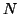subjects and that for each subject,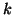, the preprocessed FMRI data is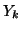(a vector of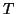time points), the design matrix is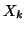and the parameter estimates are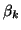(for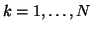). The two-level model for this experiment is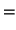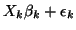(1)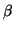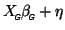(2)

where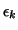specifies the single-subject residuals,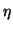specifies the residuals of the group activation (parameter) scores, and where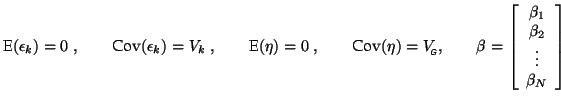denotes the combined first-level parameter estimates of the whole group, assembled into a single vector,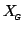is the group-level design matrix (e.g. separating controls from normals) and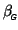is the final vector of group-level parameters.

Christian Beckmann 2003-07-16